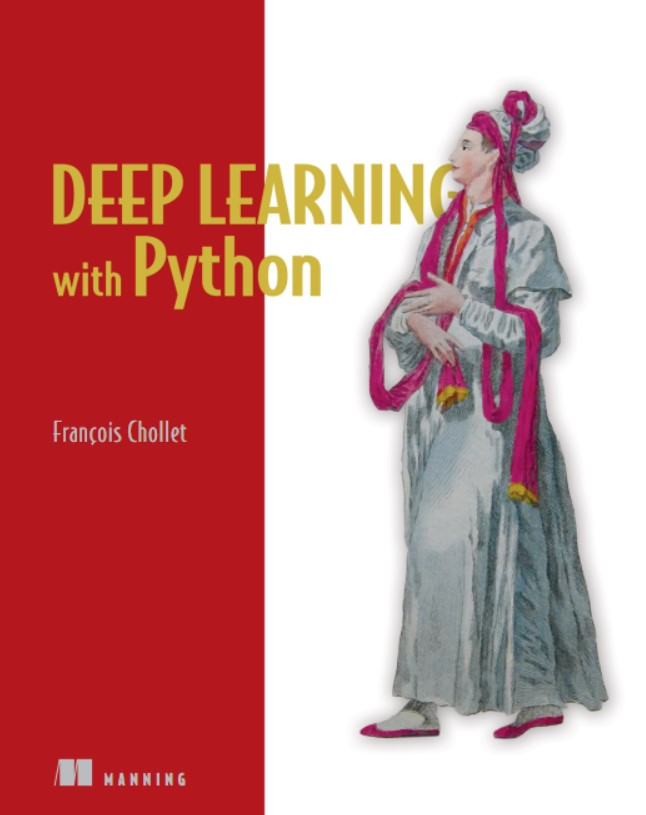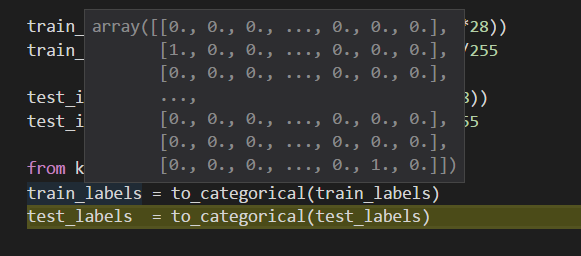#DL_WITH_PY系统学习（第2章）

0
0
01. 云栖社区>
2. 博客>
3. 正文

## DL_WITH_PY系统学习（第2章）1、第一个dl例子；
2、tensor和tensor操作；
3、DL如何通过逆向传播和梯度下降达到学习目的。

2.1 输入数据集的格式
from keras.datasets import mnist
(train_images,train_labels),(test_images,test_labels) = mnist.load_data()

print('train_image_shape',train_images.shape)
print('train_labels_len',len(train_labels))
print('train_labels',train_labels)

train_image_shape (60000, 28, 28)
train_labels_len 60000
train_labels [5 0 4 ... 5 6 8]
2.2训练网络结构
network = models.Sequential()
network.add(layers.Dense(512,activation = 'relu',input_shape = (28*28,)))
network.add(layers.Dense(10,activation='softmax'))
layers(层）是训练网络的最基本组成部分，它读入数据，输出对于结果来说更有用的结果。

2.3编译模型
network.compile(optimizer='rmaprop',loss='categorical_crossentropy',metrics=['accuracy'])

2.4处理数据集

train_images = train_images.reshape((60000,28*28))
train_images = train_images.astype('float32')/255

test_images = test_images.reshape((10000,28*28))
test_images = test_images.astype('float32')/255

2.5进行one_hot处理
from keras.utils import to_categorical
train_labels = to_categorical(train_labels)
test_labels = to_categorical(test_labels)2.6 现在就尝试训练

network.fit(train_images,train_labels,epochs=5,batch_size=128)

rain_images 是数量*（长*宽）

train_labes 是onehot格式。这两个部分首先要清楚。

test_loss,test_acc = network.evaluate(test_images,test_labels)

2.7 什么是tensor

np.array(12)就是一个0D tensor
np.array([12,3,6,14])一个列表，就是一个1D tensor
np.array([12,3,6,14],
[6,79,35,1],
[7,80,4,36,2] )

2.8  tensor的组成

import matplotlib.pyplot as plt
digit = train_images
plt.imshow(digit,cmap=plt.cm.binary)
plt.show()

2.9实际情况下的图像存储

+ 关注